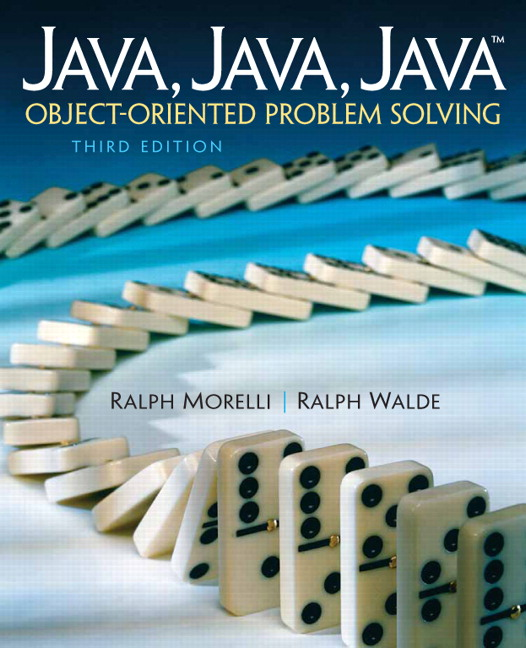# Java, Java, Java: Object-Oriented Problem Solving, 2022E

## Section3.11Drawing Lines and Defining Graphical Methods (Optional)

We used a Graphics object in the previous chapter to draw rectangles and ovals in a JFrame window. The Graphics class also possesses a method for drawing a line segment. Problems involving drawing pictures in an JFrame window using a series of line segments can be a source of examples of defining useful methods and also of making good use of loops.
The Graphics class has a public instance method with the header:
public  void  drawLine(int x1, int y1, int x2, int y2)

The method call g.drawLine(x1, y1, x2, y2) draws a line from the point $$(x1,y1)$$ to $$(x2,y2)$$ where $$(x,y)$$ refers to a point that is $$x$$ pixels from the left edge of the area that g is drawing in and $$y$$ pixels from the top edge. Thus g.drawLine(10, 10, 10, 60) draws a vertical line segment that is 50 pixels long and is 10 pixels from the left edge of the drawing area, that is, a line segment from the point $$(10,10)$$ to the point $$(10,60)\text{.}$$
Consider the problem of creating an Swing program with a method called drawSticks() to draw any specified number of vertical line segments. This method might be useful for an graphical user interface to the OneRowNim game to draw the number of sticks at a given point in a game. Suppose that this method must have an int parameter to specify the number of vertical lines to draw and two int parameters to specify the location of the top endpoint of the left most line segment. The drawSticks() method will need to use a Graphics object connected to the JFrame window for drawing the line segment. The only such Graphics object available is the parameter in the paint() method of the Canvas. Thus the method must have a Graphics parameter and it will be called in the paint() method using the Graphics object there as an argument. Thus the header of the method should look like:
public void drawSticks(Graphics g,int x,int y,int num)

The body of the method could use a while loop with a counter variable k to draw num lines. The counter k is initialized to 0 before the loop and incremented at the end of the loop. The loop runs as long as k < num.
public void drawSticks(Graphics g, int x, int y, int num)
{
int k = 0;
while (k < num)
{   g.drawLine(x, y, x, y + 50);
x = x + 10;
k = k + 1;
} // while
} // drawSticks()


### Activity3.11.1.

Run the program below listed in Listing 3.11.1 and DrawSticks on repl. Can you change the number of lines it draws?
Note that the body of drawSticks() uses a while-loop to draw the lines and declares and initializes a local variable to zero to use for counting the number of lines drawn. The statement g.drawLine(x, y, x, y + 50); draws a vertical line which is $$50$$ pixels long. Increasing the value of $$x$$ by $$10$$ each time through the loop moves the next line $$10$$ pixels to the right.
The first call to drawSticks() in the paint() method draws $$12$$ lines with $$(25,25)$$ the top point of the left-most line. The second call to drawSticks() will draw $$7$$ cyan sticks $$100$$ pixels lower. Note that changing the color of g before passing it as an argument to drawSticks() changes the drawing color.
An image of the DrawSticksCanvas as it appears in a window is shown in Figure 3.11.2.